# RD Sharma Solutions for Class 6 Maths Chapter 2: Playing with Numbers Exercise 2.1

## RD Sharma Solutions for Class 6 Maths Exercise 2.1 PDF

The primary objective of preparing solutions is to help students speed up their exam preparation. Understanding the concepts which are covered in Class 6 Mathematics is crucial as they are also continued in higher classes. Students can improve their problem solving and logical thinking abilities by practising textbook questions. The subject experts at BYJU’S create accurate solutions based on CBSE syllabus and guidelines. The solutions are available in PDF format which can be accessed easily by the students. RD Sharma Solutions for Class 6 Maths Chapter 2 Playing with Numbers Exercise 2.1 are provided here.

## RD Sharma Solutions for Class 6 Maths Chapter 2: Playing with Numbers Exercise 2.1 Download PDF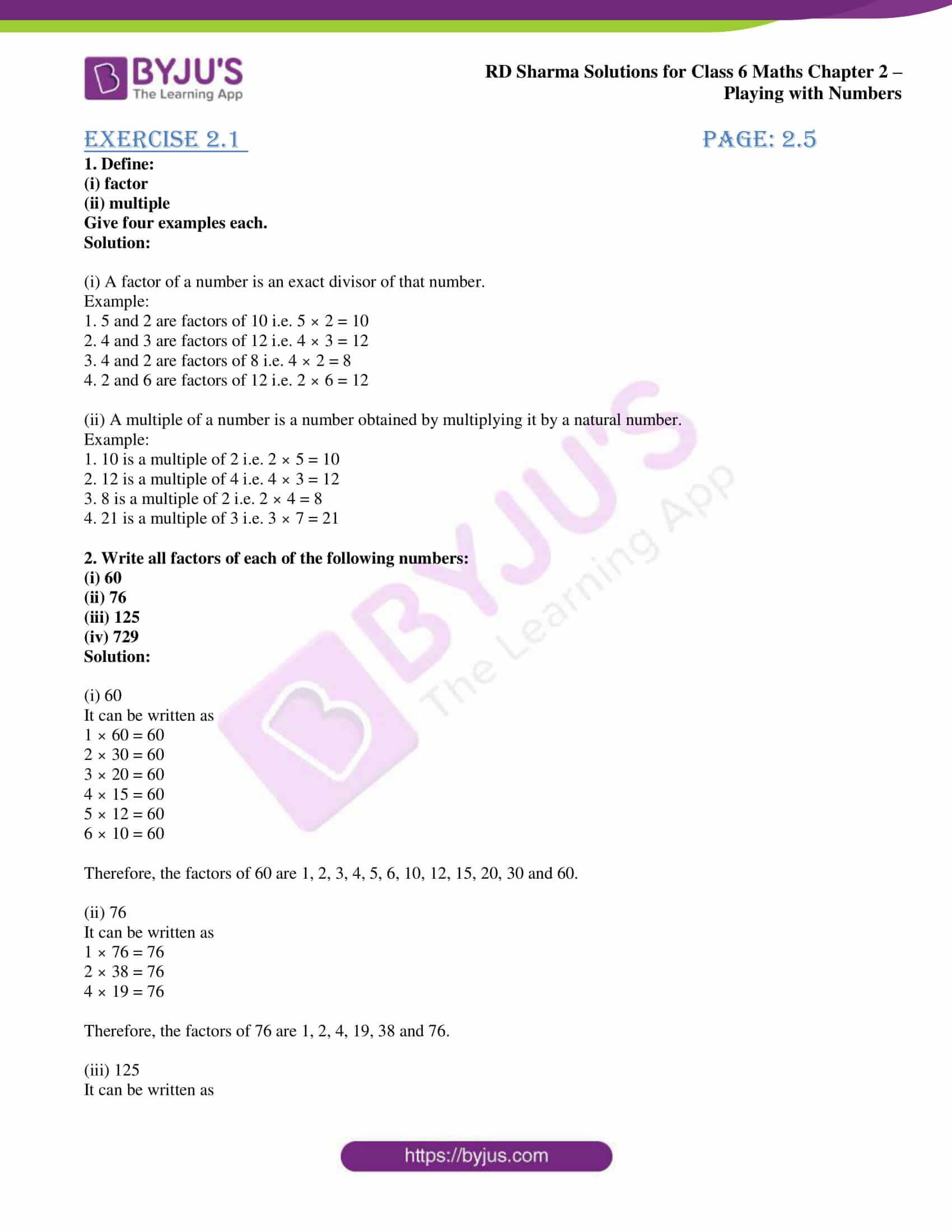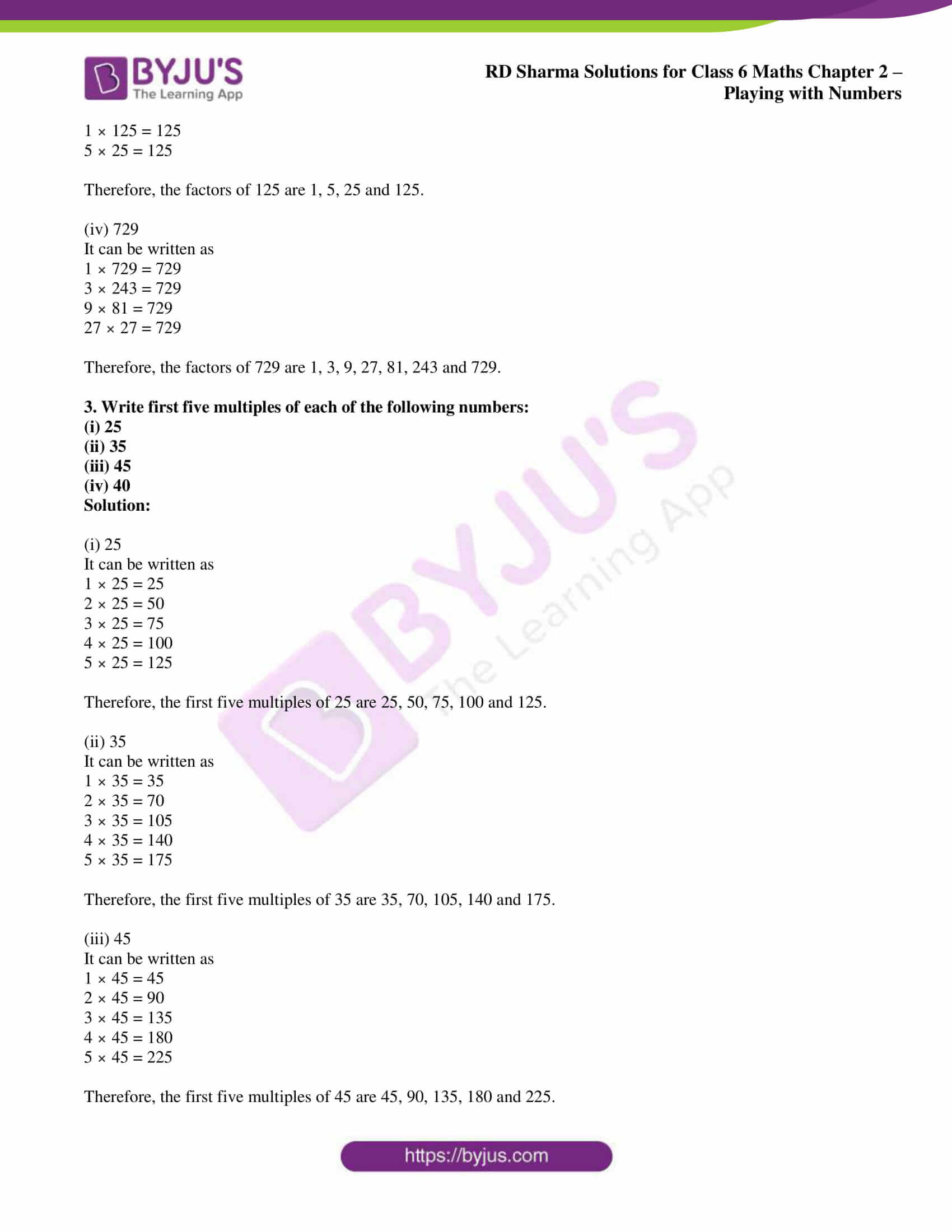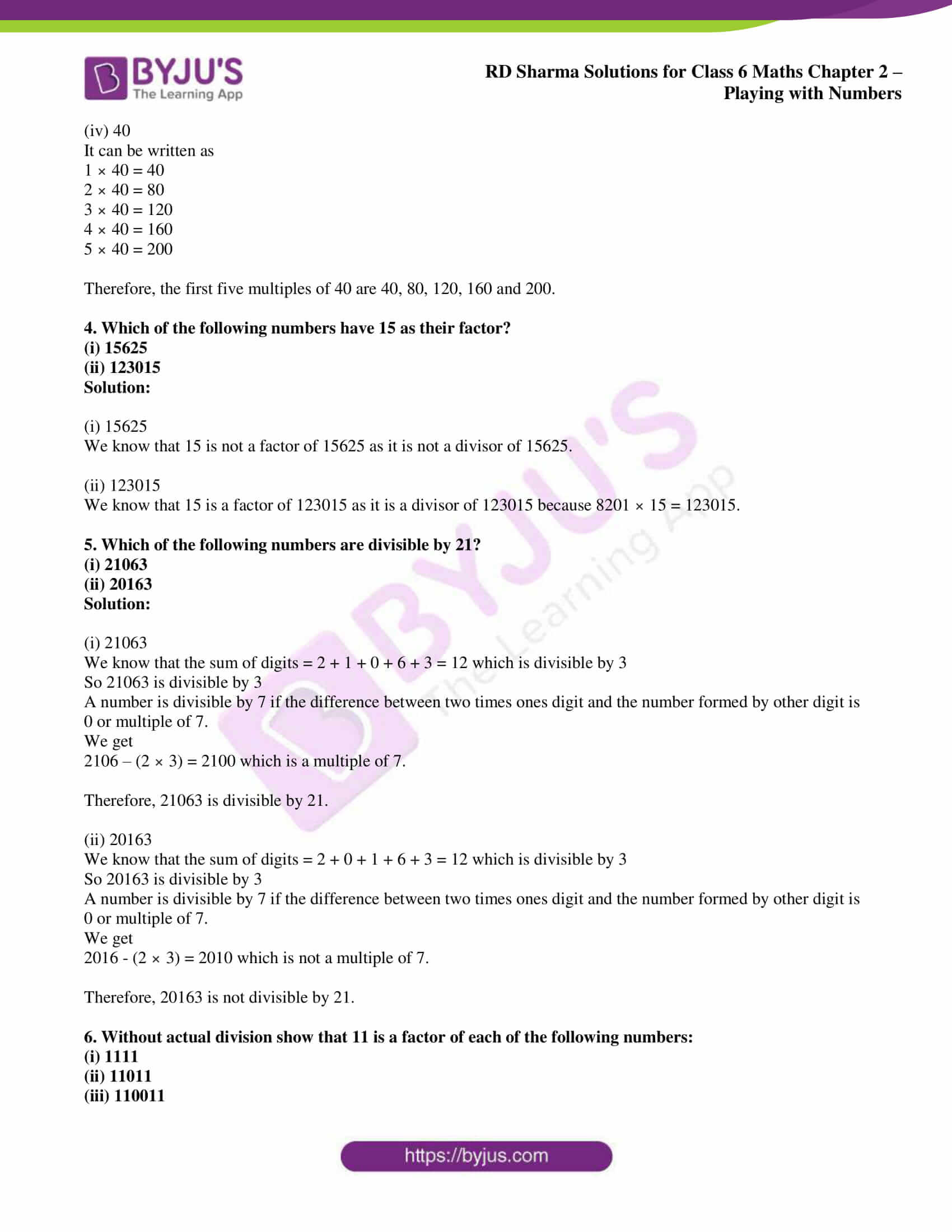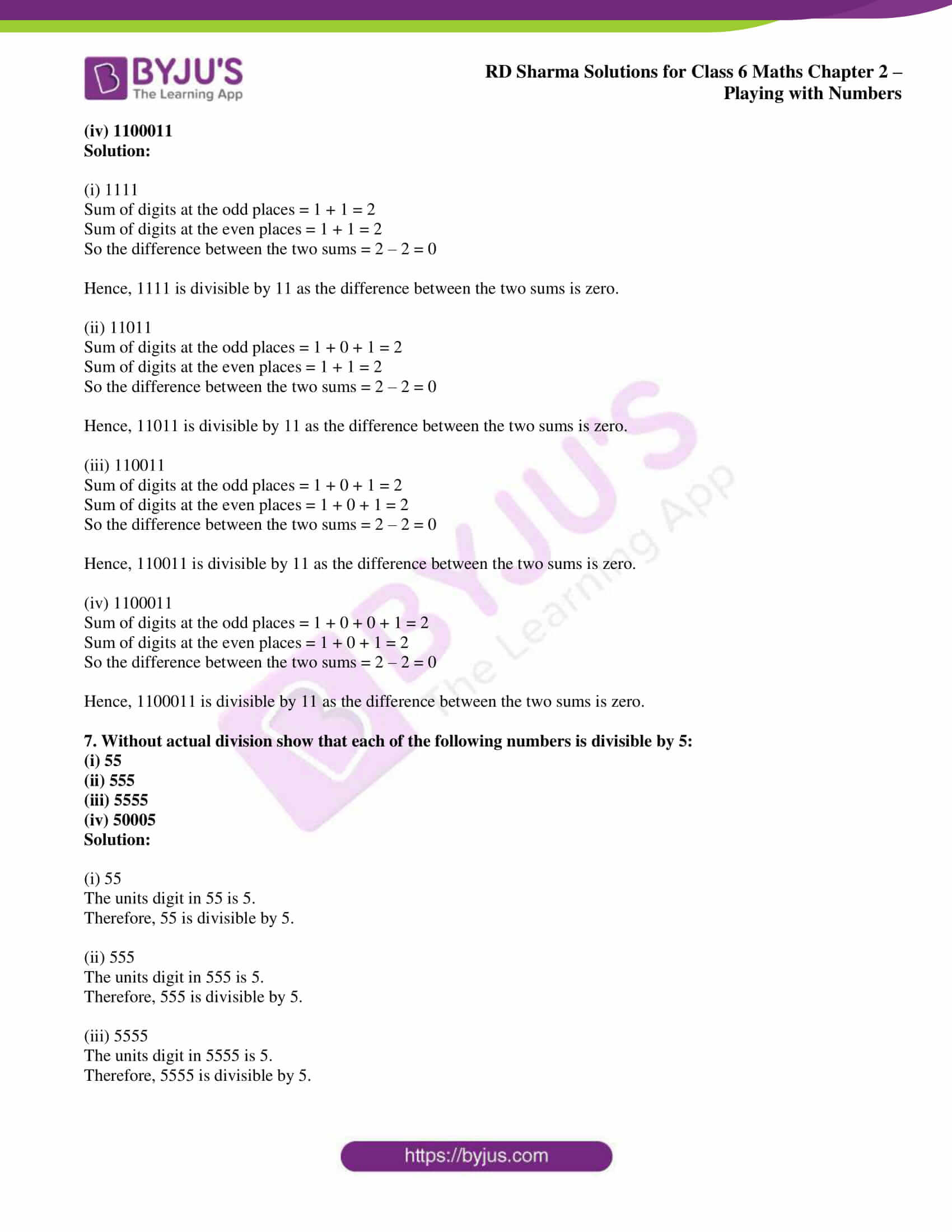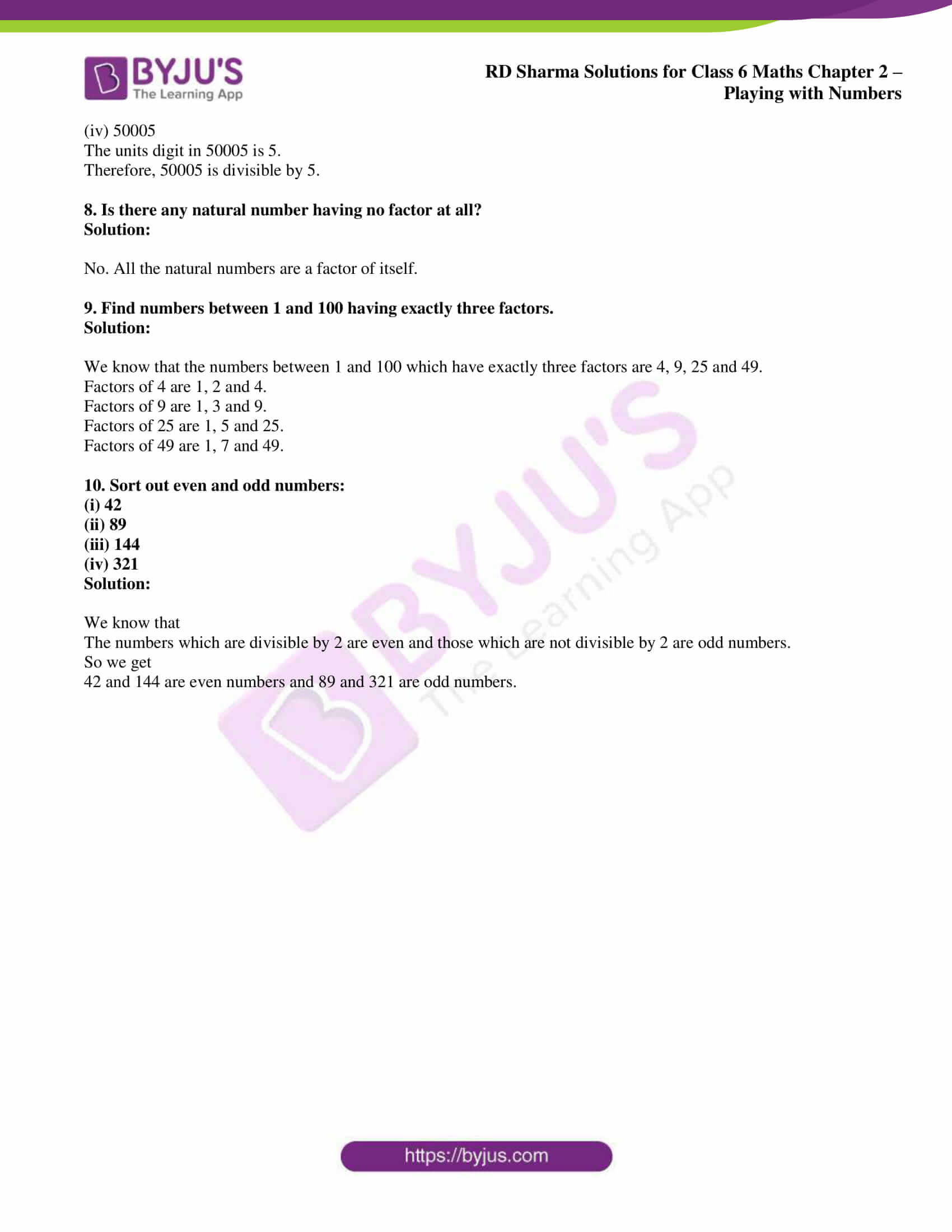## Access answers to Maths RD Sharma Solutions for Class 6 Chapter 2: Playing with Numbers Exercise 2.1

1. Define:

(i) factor

(ii) multiple

Give four examples each.

Solution:

(i) A factor of a number is an exact divisor of that number.

Example:

1. 5 and 2 are factors of 10 i.e. 5 × 2 = 10

2. 4 and 3 are factors of 12 i.e. 4 × 3 = 12

3. 4 and 2 are factors of 8 i.e. 4 × 2 = 8

4. 2 and 6 are factors of 12 i.e. 2 × 6 = 12

(ii) A multiple of a number is a number obtained by multiplying it by a natural number.

Example:

1. 10 is a multiple of 2 i.e. 2 × 5 = 10

2. 12 is a multiple of 4 i.e. 4 × 3 = 12

3. 8 is a multiple of 2 i.e. 2 × 4 = 8

4. 21 is a multiple of 3 i.e. 3 × 7 = 21

2. Write all factors of each of the following numbers:

(i) 60

(ii) 76

(iii) 125

(iv) 729

Solution:

(i) 60

It can be written as

1 × 60 = 60

2 × 30 = 60

3 × 20 = 60

4 × 15 = 60

5 × 12 = 60

6 × 10 = 60

Therefore, the factors of 60 are 1, 2, 3, 4, 5, 6, 10, 12, 15, 20, 30 and 60.

(ii) 76

It can be written as

1 × 76 = 76

2 × 38 = 76

4 × 19 = 76

Therefore, the factors of 76 are 1, 2, 4, 19, 38 and 76.

(iii) 125

It can be written as

1 × 125 = 125

5 × 25 = 125

Therefore, the factors of 125 are 1, 5, 25 and 125.

(iv) 729

It can be written as

1 × 729 = 729

3 × 243 = 729

9 × 81 = 729

27 × 27 = 729

Therefore, the factors of 729 are 1, 3, 9, 27, 81, 243 and 729.

3. Write first five multiples of each of the following numbers:

(i) 25

(ii) 35

(iii) 45

(iv) 40

Solution:

(i) 25

It can be written as

1 × 25 = 25

2 × 25 = 50

3 × 25 = 75

4 × 25 = 100

5 × 25 = 125

Therefore, the first five multiples of 25 are 25, 50, 75, 100 and 125.

(ii) 35

It can be written as

1 × 35 = 35

2 × 35 = 70

3 × 35 = 105

4 × 35 = 140

5 × 35 = 175

Therefore, the first five multiples of 35 are 35, 70, 105, 140 and 175.

(iii) 45

It can be written as

1 × 45 = 45

2 × 45 = 90

3 × 45 = 135

4 × 45 = 180

5 × 45 = 225

Therefore, the first five multiples of 45 are 45, 90, 135, 180 and 225.

(iv) 40

It can be written as

1 × 40 = 40

2 × 40 = 80

3 × 40 = 120

4 × 40 = 160

5 × 40 = 200

Therefore, the first five multiples of 40 are 40, 80, 120, 160 and 200.

4. Which of the following numbers have 15 as their factor?

(i) 15625

(ii) 123015

Solution:

(i) 15625

We know that 15 is not a factor of 15625 as it is not a divisor of 15625.

(ii) 123015

We know that 15 is a factor of 123015 as it is a divisor of 123015 because 8201 × 15 = 123015.

5. Which of the following numbers are divisible by 21?

(i) 21063

(ii) 20163

Solution:

(i) 21063

We know that the sum of digits = 2 + 1 + 0 + 6 + 3 = 12 which is divisible by 3

So 21063 is divisible by 3

A number is divisible by 7 if the difference between two times ones digit and the number formed by other digit is 0 or multiple of 7.

We get

2106 – (2 × 3) = 2100 which is a multiple of 7.

Therefore, 21063 is divisible by 21.

(ii) 20163

We know that the sum of digits = 2 + 0 + 1 + 6 + 3 = 12 which is divisible by 3

So 20163 is divisible by 3

A number is divisible by 7 if the difference between two times ones digit and the number formed by other digit is 0 or multiple of 7.

We get

2016 – (2 × 3) = 2010 which is not a multiple of 7.

Therefore, 20163 is not divisible by 21.

6. Without actual division show that 11 is a factor of each of the following numbers:

(i) 1111

(ii) 11011

(iii) 110011

(iv) 1100011

Solution:

(i) 1111

Sum of digits at the odd places = 1 + 1 = 2

Sum of digits at the even places = 1 + 1 = 2

So the difference between the two sums = 2 – 2 = 0

Hence, 1111 is divisible by 11 as the difference between the two sums is zero.

(ii) 11011

Sum of digits at the odd places = 1 + 0 + 1 = 2

Sum of digits at the even places = 1 + 1 = 2

So the difference between the two sums = 2 – 2 = 0

Hence, 11011 is divisible by 11 as the difference between the two sums is zero.

(iii) 110011

Sum of digits at the odd places = 1 + 0 + 1 = 2

Sum of digits at the even places = 1 + 0 + 1 = 2

So the difference between the two sums = 2 – 2 = 0

Hence, 110011 is divisible by 11 as the difference between the two sums is zero.

(iv) 1100011

Sum of digits at the odd places = 1 + 0 + 0 + 1 = 2

Sum of digits at the even places = 1 + 0 + 1 = 2

So the difference between the two sums = 2 – 2 = 0

Hence, 1100011 is divisible by 11 as the difference between the two sums is zero.

7. Without actual division show that each of the following numbers is divisible by 5:

(i) 55

(ii) 555

(iii) 5555

(iv) 50005

Solution:

(i) 55

The units digit in 55 is 5.

Therefore, 55 is divisible by 5.

(ii) 555

The units digit in 555 is 5.

Therefore, 555 is divisible by 5.

(iii) 5555

The units digit in 5555 is 5.

Therefore, 5555 is divisible by 5.

(iv) 50005

The units digit in 50005 is 5.

Therefore, 50005 is divisible by 5.

8. Is there any natural number having no factor at all?

Solution:

No. All the natural numbers are a factor of itself.

9. Find numbers between 1 and 100 having exactly three factors.

Solution:

We know that the numbers between 1 and 100 which have exactly three factors are 4, 9, 25 and 49.

Factors of 4 are 1, 2 and 4.

Factors of 9 are 1, 3 and 9.

Factors of 25 are 1, 5 and 25.

Factors of 49 are 1, 7 and 49.

10. Sort out even and odd numbers:

(i) 42

(ii) 89

(iii) 144

(iv) 321

Solution:

We know that

The numbers which are divisible by 2 are even and those which are not divisible by 2 are odd numbers.

So we get

42 and 144 are even numbers and 89 and 321 are odd numbers.

### RD Sharma Solutions for Class 6 Maths Chapter 2 – Playing with Numbers Exercise 2.1

RD Sharma Solutions Class 6 Maths Chapter 2 Playing with Numbers Exercise 2.1 explains concepts like Factors and Multiples along with the methods and formulas which are used in solving exercise problems.

### Key features of RD Sharma Solutions for Class 6 Maths Chapter 2: Playing with Numbers Exercise 2.1

• RD Sharma Solutions help students to solve exercise wise problems and revise the entire syllabus.
• The students gain a better knowledge about the concepts covered in each exercise along with the important topics.
• Solutions PDF contains answers for all the exercise wise problems, which are solved in a comprehensive manner.
• Self analysis is made easier for the students, which helps them in focusing on their areas of weaknesses.